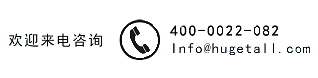# 迈耶公式及其表示

Meyer（1908）Pa之间建立了以下经验关系

（3.97）$P=c{a}^{m}.$

（3.98）$P=K{a}^{m}/{D}^{m-2}\phantom{\rule{1em}{0ex}}\text{or}\phantom{\rule{1em}{0ex}}P/{a}^{2}=K{\left(a/D\right)}^{m-2},$

（3.99）$l\left(D,P,t\right)={\left(D/{D}_{1}\right)}^{\kappa /\left(2+\kappa \right)}{\left(P/{P}_{1}\right)}^{1/\left(2+\kappa \right)}l\left({D}_{1},{P}_{1},t\right),$